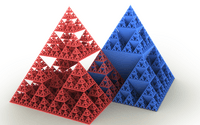# Sierpinski triangle facts for kids

Kids Encyclopedia Facts

A Sierpinski triangle or Sierpinski triangle gasket is a fractal resulting from doing the following:

• Remove center part.
• Do the same for the three largest equilateral triangles left in this one.

If this is done, the first few steps will look like this:

If this is done an infinite number of times, its area will be 0.

They can also be 3D:## Images for kidsSierpinski triangle Facts for Kids. Kiddle Encyclopedia.